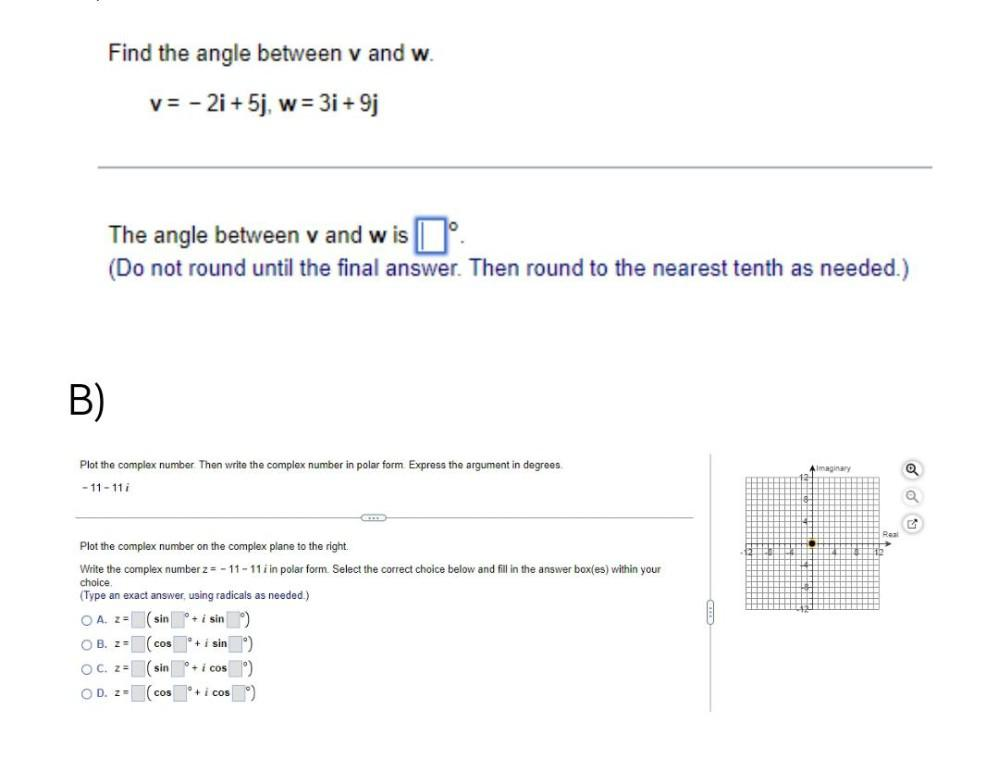Question:

# Find the angle between v and w. v = -2i+ 5j, w = 3i+9j The

Last updated: 7/30/2022Find the angle between v and w. v = -2i+ 5j, w = 3i+9j The angle between v and w is ____º. (Do not round until the final answer. Then round to the nearest tenth as needed.) Plot the complex number. Then write the complex number in polar form. Express the argument in degrees. -11-11i Plot the complex number on the complex plane to the right. Write the complex number z=-11-11i in polar form. Select the correct choice below and fill in the answer box(es) within your choice. (Type an exact answer, using radicals as needed.)